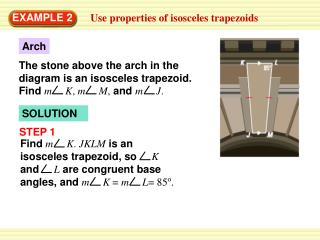DownloadDownload PresentationEXAMPLE 2

# EXAMPLE 2

Download Presentation## EXAMPLE 2

- - - - - - - - - - - - - - - - - - - - - - - - - - - E N D - - - - - - - - - - - - - - - - - - - - - - - - - - -
##### Presentation Transcript

1. Arch The stone above the arch in the diagram is an isosceles trapezoid. Find mK, mM, and mJ. STEP 1 Find mK. JKLMis an isosceles trapezoid, so Kand Lare congruent base angles, and mK = mL= 85o. EXAMPLE 2 Use properties of isosceles trapezoids SOLUTION

2. STEP 2 Find m M. Because Land M are consecutive interior angles formed by LMintersecting two parallel lines,they are supplementary. So,mM = 180o – 85o = 95o. STEP 3 Find mJ. Because J and M are a pair of base angles, they are congruent, and mJ = mM=95o. ANSWER So, mJ = 95o, m K = 85o, and m M = 95o. EXAMPLE 2 Use properties of isosceles trapezoids

3. In the diagram,MNis the midsegment of trapezoidPQRS. FindMN. SOLUTION Use Theorem 8.17 to findMN. 1 = (12+ 28) 2 1 MN (PQ + SR) = 2 ANSWER The length MNis 20 inches. EXAMPLE 3 Use the midsegment of a trapezoid Apply Theorem 8.17. Substitute 12 for PQand 28 for XU. = 20 Simplify.

4. 3. If EG = FH, is trapezoid EFGHisosceles? Explain. ANSWER Yes, trapezoid EFGH is isosceles, if and only if its diagonals are congruent. As, it is given its diagonals are congruent, therefore by theorem 8.16 the trapezoid is isosceles. for Examples 2 and 3 GUIDED PRACTICE In Exercises 3 and 4, use the diagram of trapezoidEFGH.

5. 4. If mHEF = 70o and mFGH =110o, is trapezoid EFGHisosceles?Explain. ANSWER G and F are consecutive interior angles as EF HG. Because FGH=110°, therefore EFG=70° as they are supplementary angles. BHG=110°, as the sum of a quadrilateral is 360° . The base angles are congruent that is, 110° each therefore, the trapezoid is isosceles by theorem 8.15. for Examples 2 and 3 GUIDED PRACTICE

6. J K 9 cm 12 cm P N ANSWER NPis the midsegment of trapezoidJKLM. x cm M L 1 5. In trapezoid JKLM, Jand M are right angles, and JK = 9cm. The length of the midsegment NPof trapezoid JKLMis 12cm. Sketch trapezoid JKLMand its midsegment. Find ML. Explain your reasoning. 2 andNP = ( JK + ML) for Examples 2 and 3 GUIDED PRACTICE

7. 1 (JK + ML) NP = 2 12 24 = 9 + x 15 = x 1 = (9 + x) 2 for Examples 2 and 3 GUIDED PRACTICE Apply Theorem 8.17. Substitute Multiply each side by 2 Simplify.